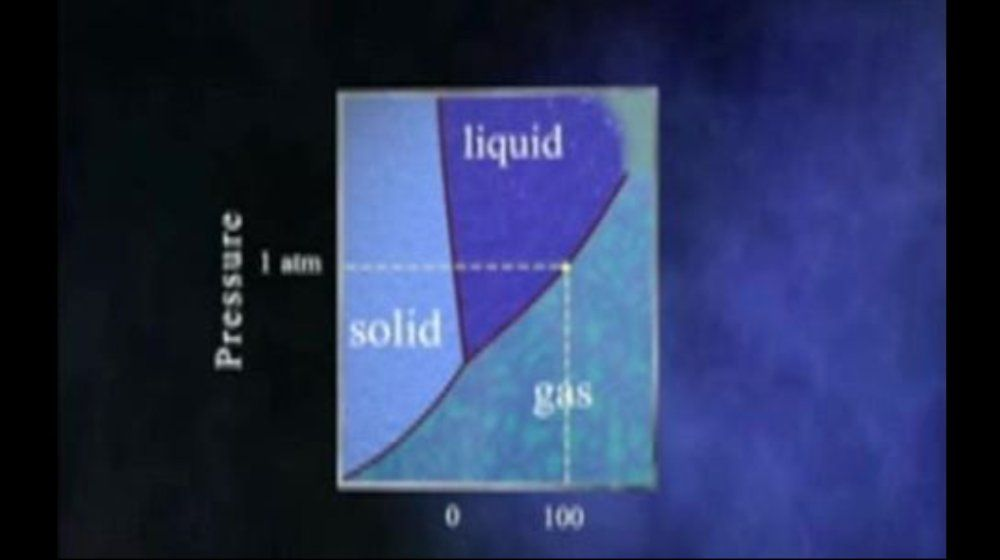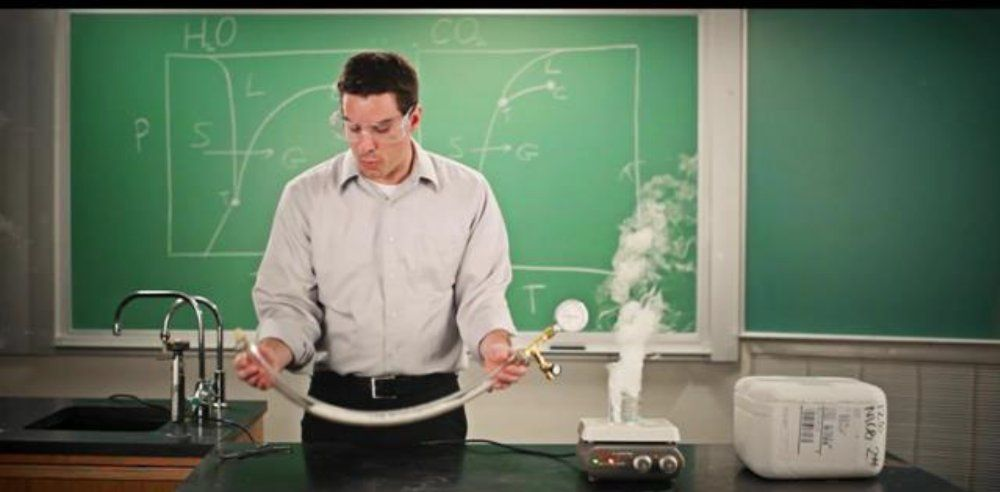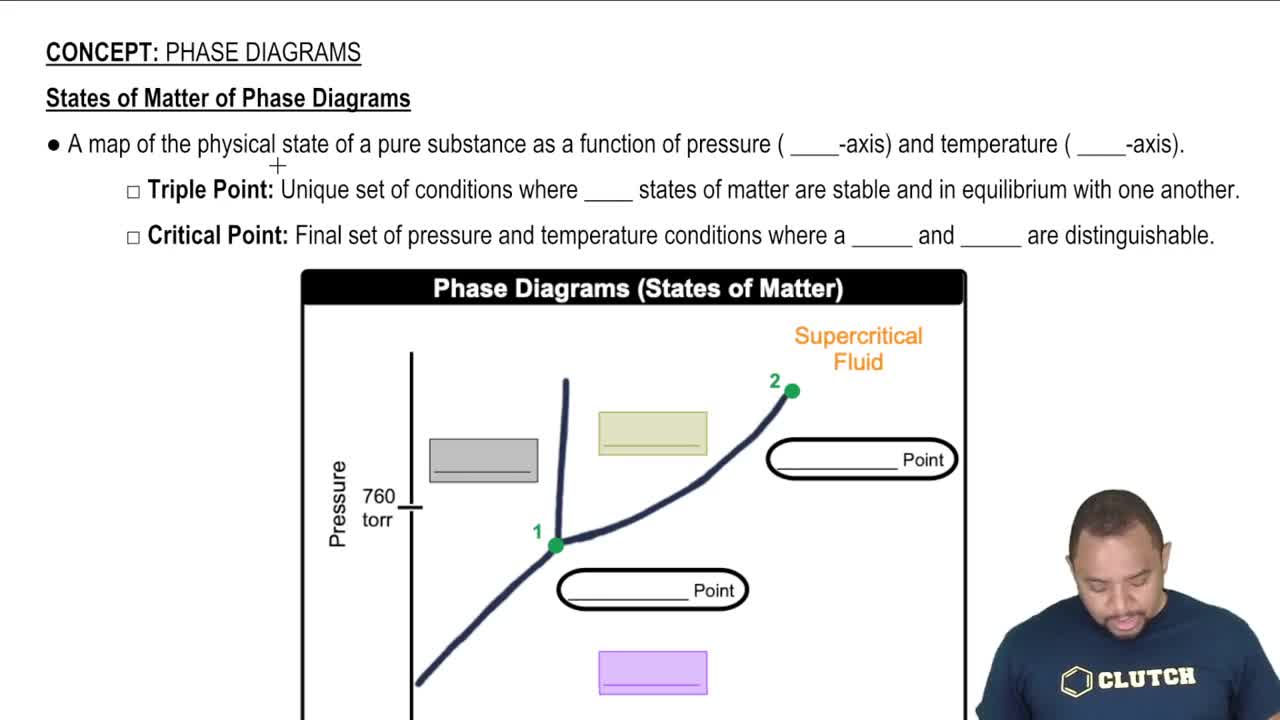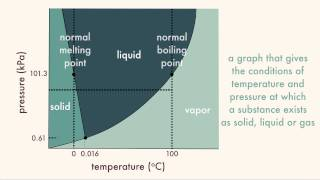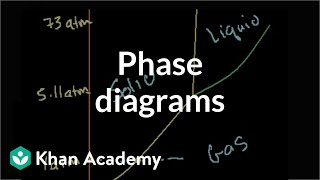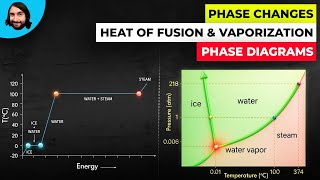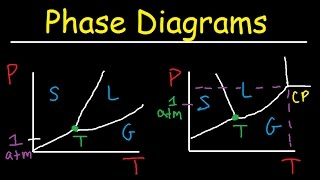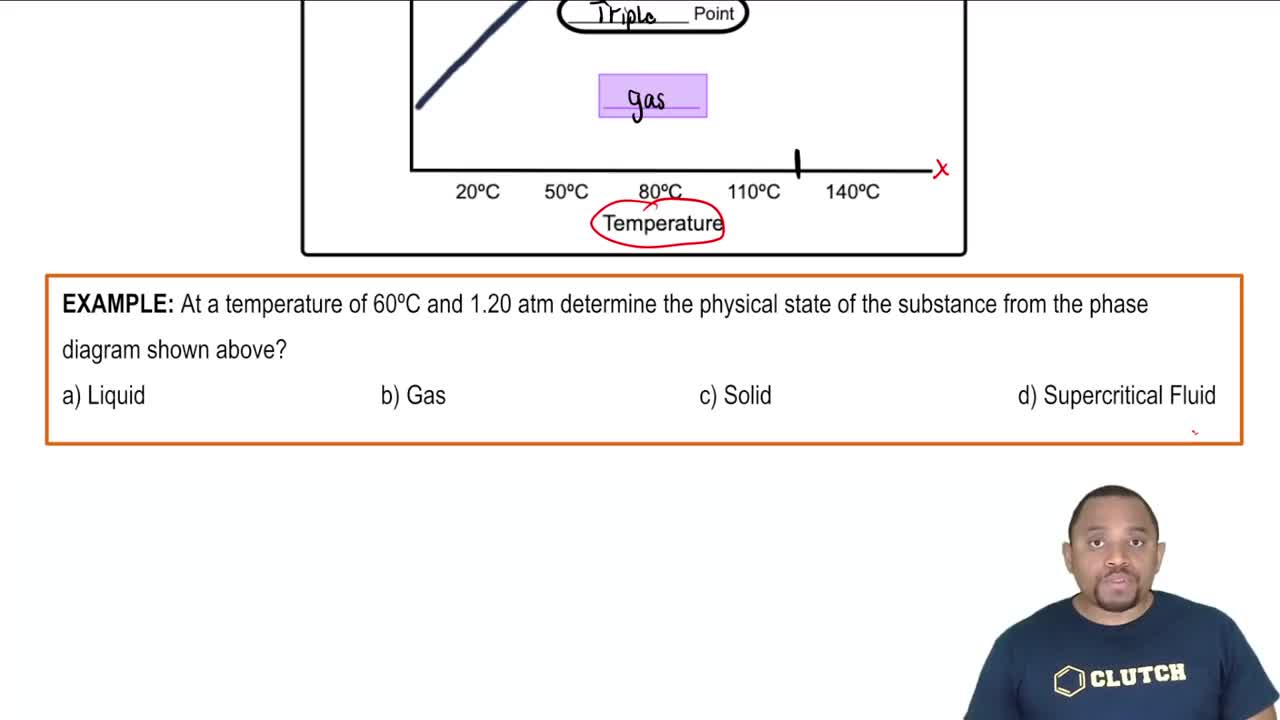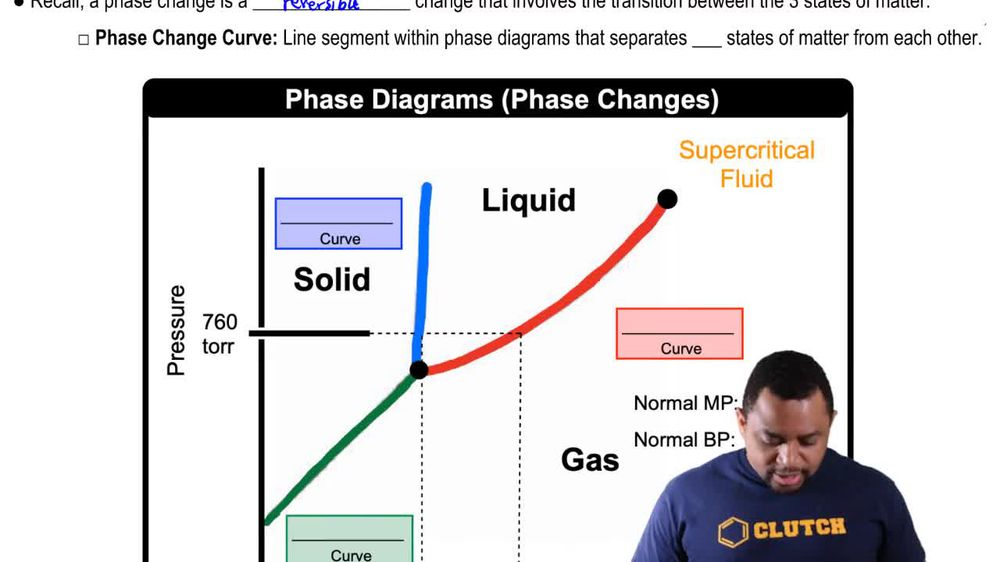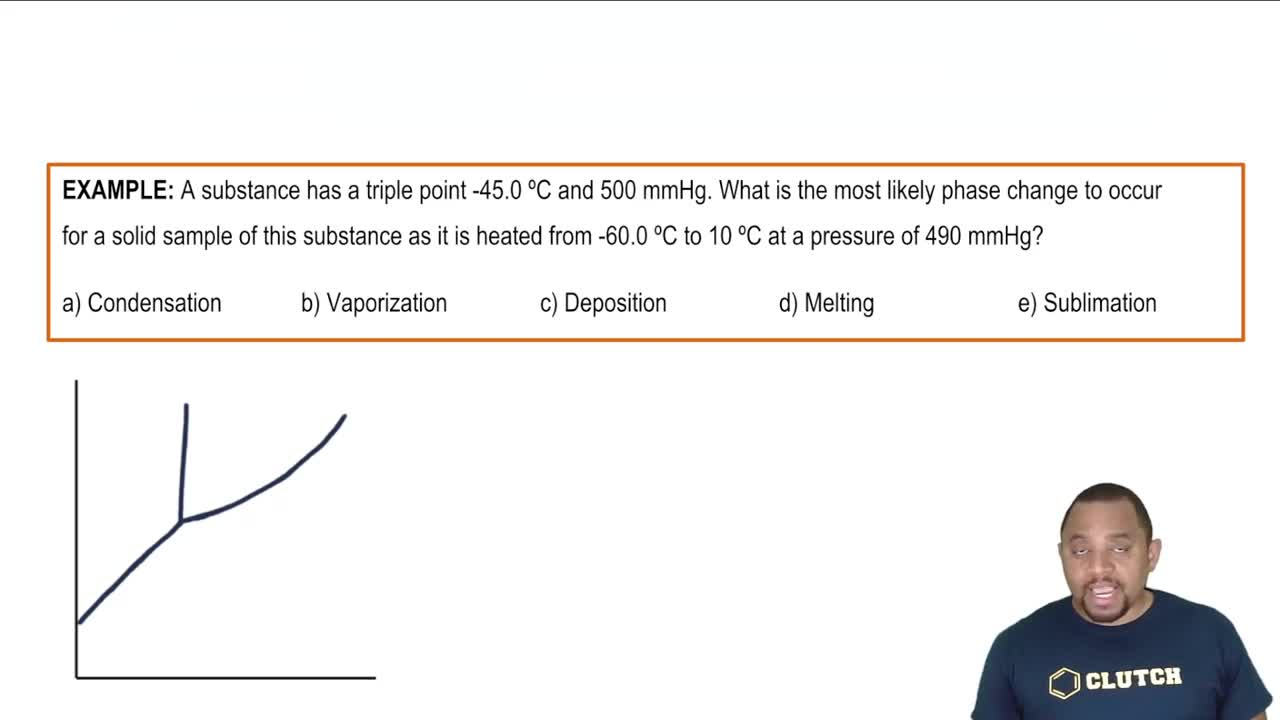Start typing, then use the up and down arrows to select an option from the list.
1. 13. Liquids, Solids & Intermolecular Forces2. Phase Diagrams# Phase Diagrams Example 1

by Jules Bruno
99 views
0
at a temperature of 65 degrees Celsius at 1.20 atmospheres determine the physical state of the substance from the phase diagram shown above. All right. So, if we take a look here at this phase diagram shown above, they're telling us we're dealing with 65 degrees Celsius, which means that somewhere between 50 degrees Celsius and 80 Let's say about 65 is around here or so. Somewhere around here. And they're telling me a pressure of 1.20 atmospheres. Remember 760 tours is equal to one atmosphere. So, if we're saying 120, that would mean that would be a pressure above this line here. Mhm. So, the pressure would fall somewhere within this region above tour. And we can clearly see that would mean that my substance would exist as a liquid. Because here are temperature. We'll line up here and our pressures somewhere within here. So that would mean that our answer would have to be option A Our substance exists as a liquid. It wouldn't be a gas, a solid or a supercritical fluid. So, again, option is our best answer